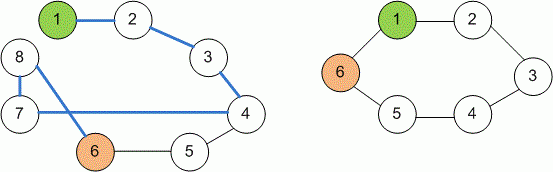Competitions

Shortest even path

Undirected unweighted graph is given. Find the shortest path between two vertices of even length.

Input

First line contains four integers: number of vertices n, number of edges m (n, m105), source s and destination d (1s, dn). Each of the next m lines contains two integers a and b describing an undirected edge (a, b).

Output

Print the shortest distance from s to d. The length of the path (number of edges in the path) must be even. If there is no path of even length between s and d, print -1.Time limit 2 seconds
Memory limit 128 MiB
Input example #1
8 8 1 6
1 2
2 3
3 4
4 5
5 6
4 7
7 8
8 6
Output example #1
6
Input example #2
6 5 1 6
1 2
2 3
3 4
4 5
5 6
Output example #2
-1
Author Mykhailo Medvediev